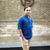# Rounding and converting raster values

1755
16
07-27-2021 07:15 AM
Labels (2)by
New Contributor III

Hello,

I'm using ArcGIS Pro 2.8.1. I have a raster layer whose values I would like to round and then convert into a corresponding value. For example, if the current value is 9.788, I would round that to 10 and convert that to 144. Is there a way to do this?

Tags (3)
1 Solution

Accepted SolutionsbyMVP Esteemed Contributor

Let me try putting it in a single expression

Expression:

``16*((Con(("RASTER" - (RoundDown("RASTER"))== 0.5),RoundUp("RASTER"),RoundUp("RASTER" - 0.5)))-1)+1``

Think Location
16 RepliesbyMVP Esteemed Contributor

A RoundUp() function of (Raster Value minus 0.5), inside a Con() function should work.

In the following example, The Raster Calculator is checking for the Round Value is equal to 10. If the argument is true it will return 144. Else it will return the original Raster Value.

``Con((RoundUp("RASTER" - 0.5)==10),144,"RASTER")``

If required, you could further add Con() condition in place of the False Value (Nested condition). The ultimate False Value, I am keeping as 0 in this example.

``Con((RoundUp("RASTER" - 0.5)==10),144,(Con((RoundUp("RASTER" - 0.5)==11),169,0)))``

Hope it helps.

Think Locationby
New Contributor III

Hello,

Thank you so much for this! Could you please clarify why 0.5 is subtracted?byMVP Esteemed Contributor

Good Question.

I couldn't find a function to do a proper Round, i.e. RoundDown values from decimal values of .0 to .499... and RoundUp Values from .5 to .99...

So deducting a 0.5 will always given me a value, which if Rounded Up, gives you the desired value.

A few examples

9.569 : 9.569-0.5=9.069: RoundUp(9.069)=10

9.0: 9.0-0.5=8.5: RoundUp(8.5)=9

9.25: 9.25-0.5=8.75: RoundUp(8.75)=9

*Just realised

The only issue is with values ending with .5 decimals.

9.5: 9.5-0.5=9.0: RoundUp(9.0)=9 (But you want 10 in this case) 😔

Alternatively, you could add 0.5 and use RoundDown() function.

Think Locationby
New Contributor III

That makes sense! What would your recommendation be in cases of 0.5 decimals?byMVP Esteemed Contributor

``Con(("RASTER" - (RoundDown("RASTER"))== 0.5),RoundUp("RASTER"),"RASTER")``

Use its output Raster as the input Raster of the expression in my first post. Like this

``Con((RoundUp("NEWRASTER" - 0.5)==10),144,0)``

You may choose to create a model in Model Builder, if this is a repetitive process.

I don't want to complicate the expression. That's why providing the solution in two steps.

Think LocationbyMVP Esteemed Contributor

Is this value the only one that you want to round?

What is the significance of rounding it to 10, then converting it to 144?

Are you trying to scale a raster's data to a new range?

... sort of retired...by
New Contributor III

There are multiple values I would like to round. In a nutshell, I have satellite data recorded in 16-day intervals, which are numbered 1-23. I want to convert these numbers to the Julian day of year (i.e. the 2nd timestep, currently labelled 2, would be converted to day 16). I need to round the numbers so I can identify which day of year it is closest to (i.e. if the value was 2.34, I would assign it to the second timestep, day 16). Please let me know if you need further clarification!byMVP Esteemed Contributor

Let me try putting it in a single expression

Expression:

``16*((Con(("RASTER" - (RoundDown("RASTER"))== 0.5),RoundUp("RASTER"),RoundUp("RASTER" - 0.5)))-1)+1``

Think Locationby
New Contributor III

Thank you both so much for catching this! JayanthaPoddar, could I ask again for you to clarify the purpose of subtracting 1?